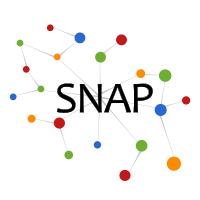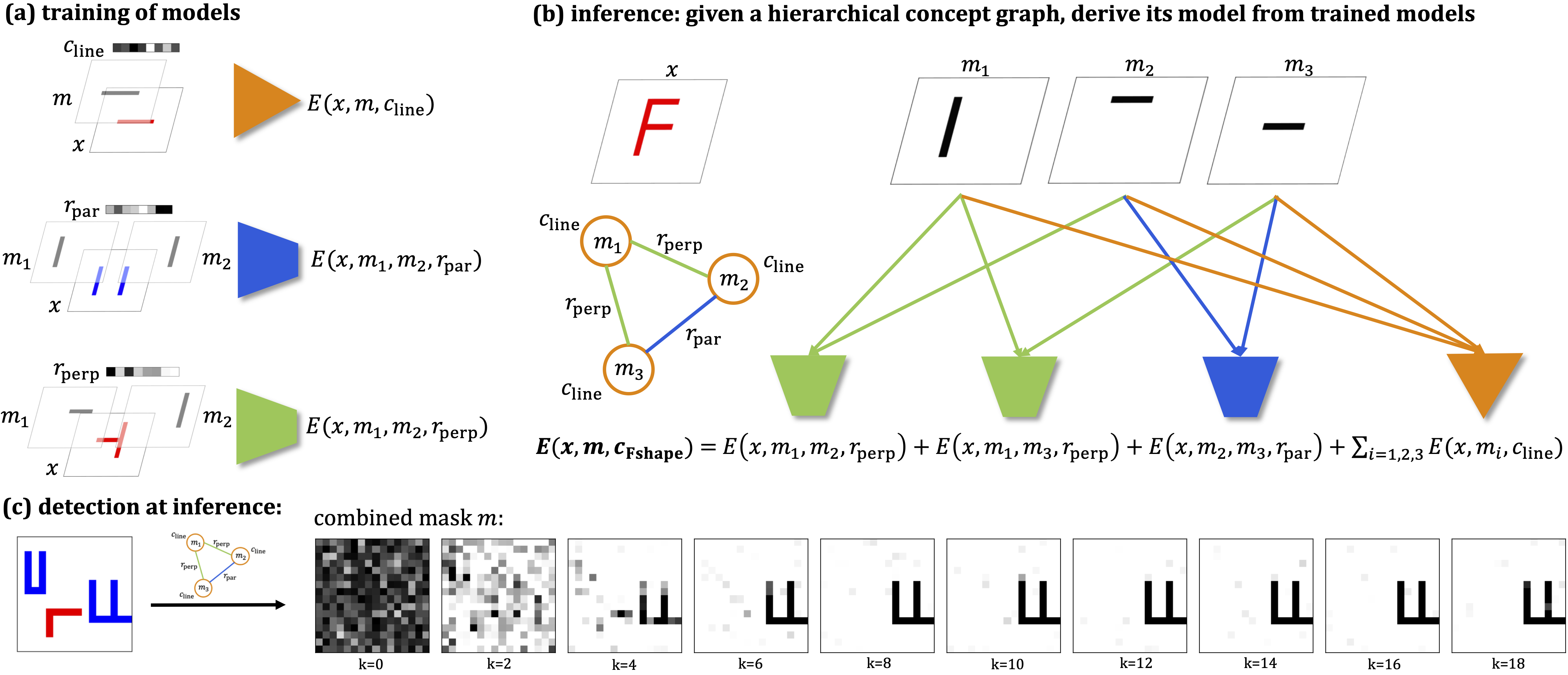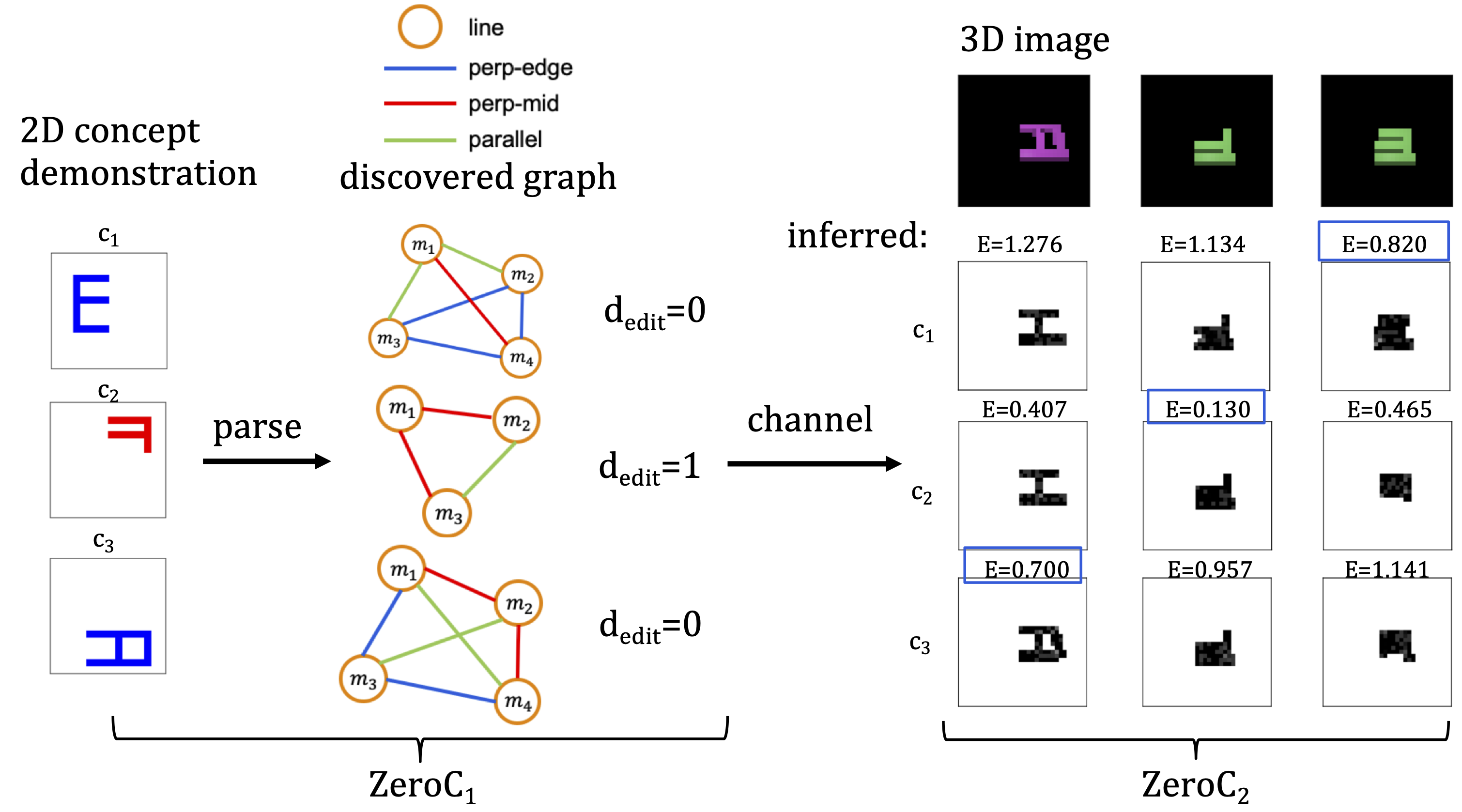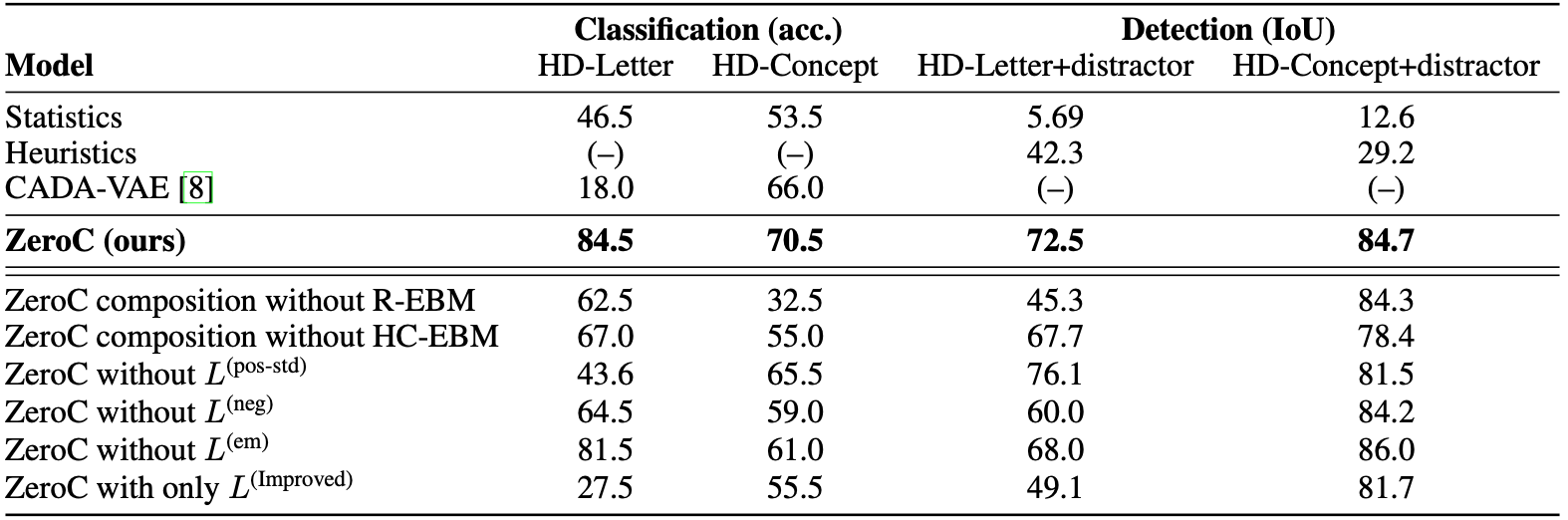# ZeroC: A Neuro-Symbolic Model for Zero-shot Concept Recognition and Acquisition at Inference Time

We introduce Zero-shot Concept Recognition and Acquisition (ZeroC), a neuro-symbolic architecture that can recognize and acquire novel concepts in a zero-shot way. ZeroC represents concepts as graphs of constituent concept models (as nodes) and their relations (as edges). For the first time, it allows acquiring new concepts, communicating its graph structure, and applying it to classification and detection tasks (even across domains) at inference time.

## Method

Humans have the remarkable ability to recognize and acquire novel visual concepts in a zero-shot manner. Given a high-level, symbolic description of a novel concept in terms of previously learned visual concepts and their relations, humans can recognize novel concepts without seeing any examples. Moreover, they can acquire new concepts by parsing and communicating symbolic structures using learned visual concepts and relations. Endowing these capabilities in machines is pivotal in improving their generalization capability at inference time.

In this work, we introduce Zero-shot Concept Recognition and Acquisition (ZeroC), a neuro-symbolic architecture that can recognize and acquire novel concepts in a zero-shot way. ZeroC represents concepts as graphs of constituent concept models (as nodes) and their relations (as edges). To allow inference time composition, we employ energy-based models (EBMs) to model concepts and relations. We design ZeroC architecture so that it allows a one-to-one mapping between a symbolic graph structure of a concept and its corresponding EBM, which for the first time, allows acquiring new concepts, communicating its graph structure, and applying it to classification and detection tasks (even across domains) at inference time. We introduce algorithms for learning and inference with ZeroC.

The following figure shows how ZeroC recognizes novel hierarchical concepts at inference time. (a) During training, it learns the models for constituent concepts, the concept "line" in this case, and relations, which are "parallel" and "perpendicular". (b) During inference, it takes the concept graph of "F" and use it to derive the model for "F" from the models of its constituents. Note that no training is performed on the hierarchical concept "F". (c) Based on the composed model of "F", it is able to discover, without any more training, the mask for the hierarchical concept "F" in the presence of distractors, even though it has only learned constituent concepts of "line" and relations of "parallel" and "perpendicular".The following figure shows how ZeroC acquires and communicates novel hierarchical concepts, even across domains, at inference time (with actual example results). ZeroC$_1$ and ZeroC$_2$ are independently trained in their respective domain (2D and 3D images). At inference, ZeroC$_1$ sees the 2D demonstration of three images showing three unseen concepts $c_1$, $c_2$, $c_3$. It first parses each image into respective concept graphs. We see that except for $c_2$ that has an edit distance $d_\text{edit}$ of 1, the others have perfect parsing. ZeroC$_1$ then sends to ZeroC$_2$ the parsed concept graphs, which ZeroC$_2$ uses to perform classification that selects which 3D image corresponds to each concept.We evaluate ZeroC on a challenging grid-world dataset which is designed to probe zero-shot concept recognition and acquisition, and demonstrate its capability. For example, for zero-shot concept recognition of hierarchical concepts, ZeroC outperforms state-of-the-art zero-shot learning method of CADA-VAE.## Code

A reference implementation of ZeroC in PyTorch will be available on GitHub.

## Datasets

The datasets used by ZeroC are included and can be generated in the code repository.

## Contributors

The following people contributed to ZeroC:
Tailin Wu
Megan Tjandrasuwita
Zhengxuan Wu
Xuelin Yang
Kevin Liu
Rok Sosič
Jure Leskovec

## References

ZeroC: A Neuro-Symbolic Model for Zero-shot Concept Recognition and Acquisition at Inference Time. T. Wu, M. Tjandrasuwita, Z. Wu, X. Yang, K. Liu, R. Sosič, J. Leskovec. NeurIPS 2022.